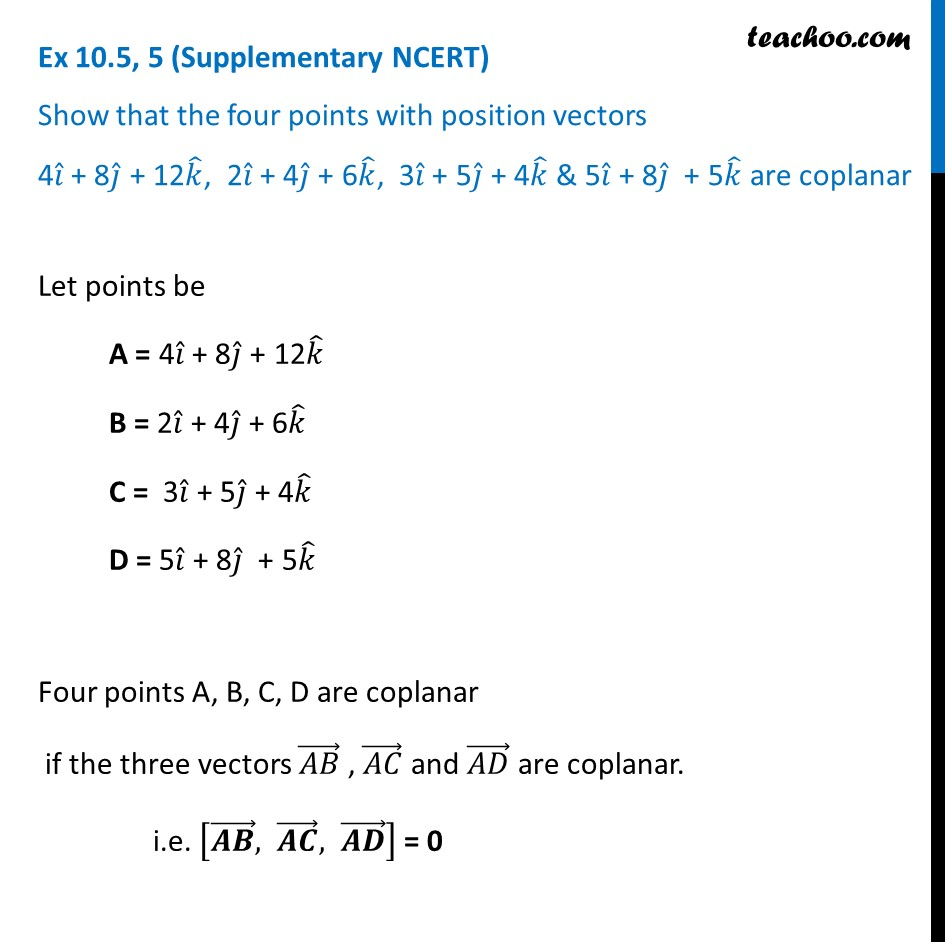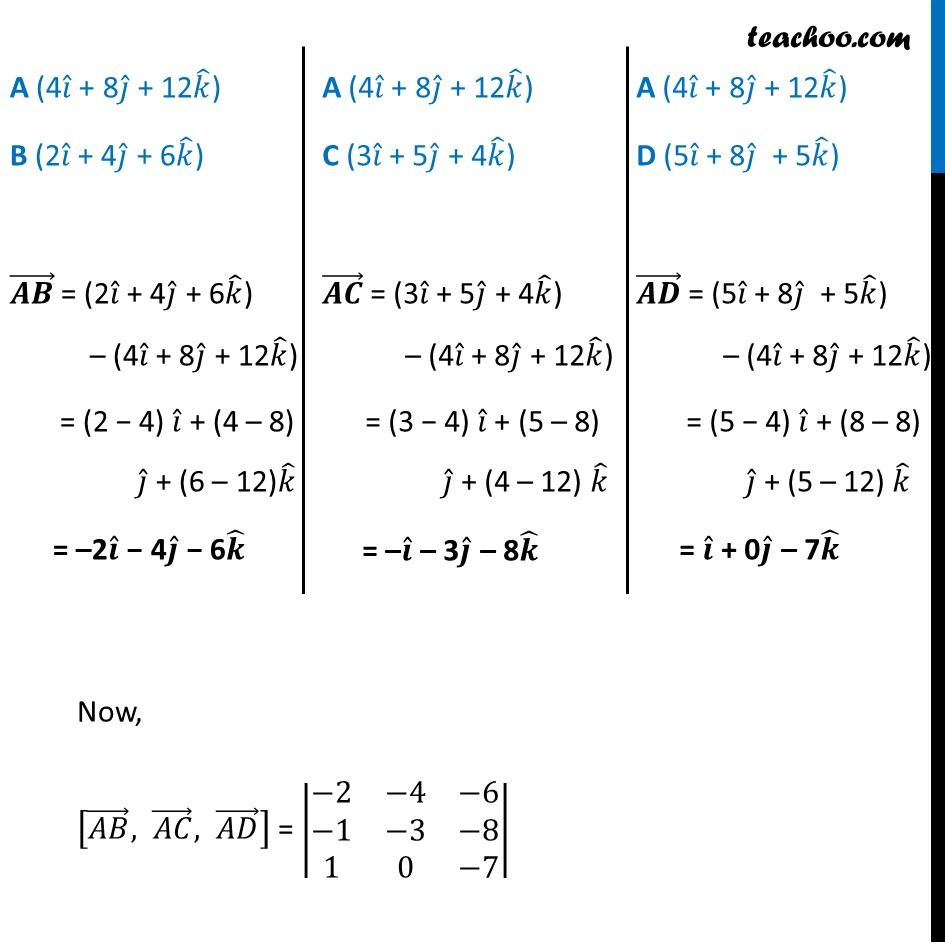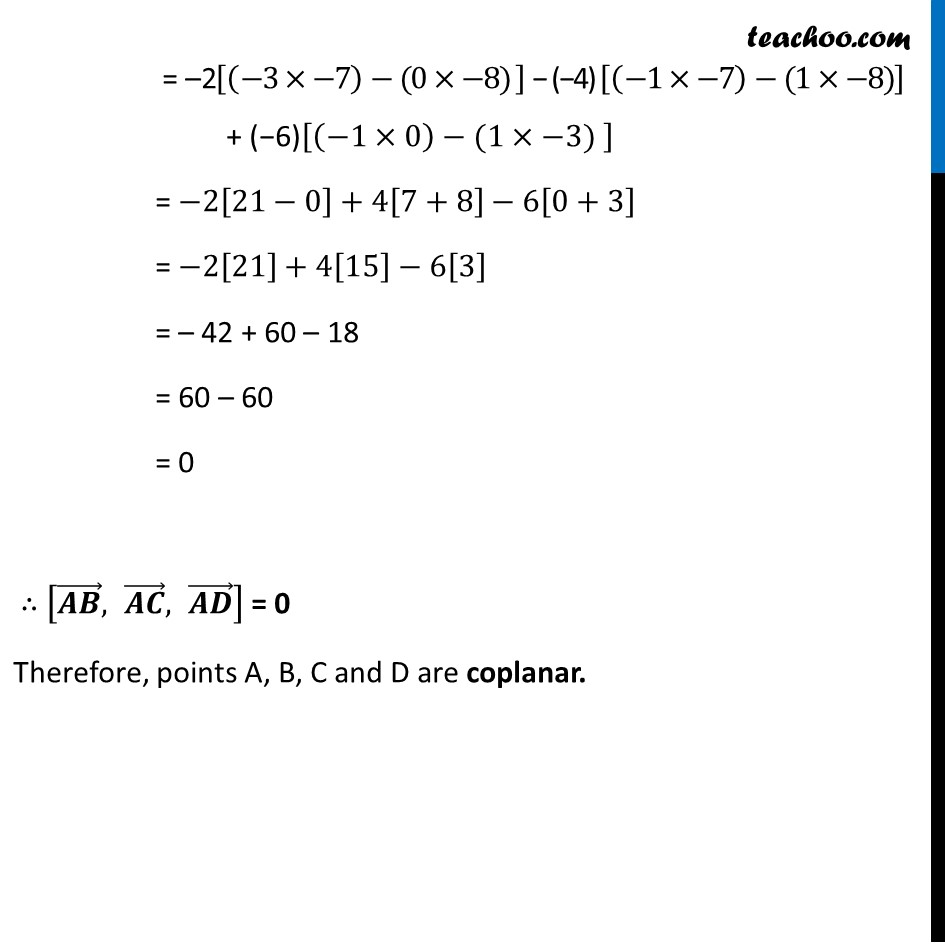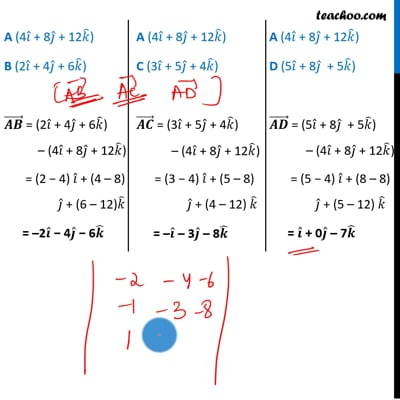Ex 10.5 (Supplementary NCERT)

Chapter 10 Class 12 Vector Algebra
Serial order wiseThis video is only available for Teachoo black users

Introducing your new favourite teacher - Teachoo Black, at only ₹83 per month

### Transcript

Ex 10.5, 5 (Supplementary NCERT) Show that the four points with position vectors 4𝑖 ̂ + 8𝑗 ̂ + 12𝑘 ̂, 2𝑖 ̂ + 4𝑗 ̂ + 6𝑘 ̂, 3𝑖 ̂ + 5𝑗 ̂ + 4𝑘 ̂ & 5𝑖 ̂ + 8𝑗 ̂ + 5𝑘 ̂ are coplanarLet points be A = 4𝑖 ̂ + 8𝑗 ̂ + 12𝑘 ̂ B = 2𝑖 ̂ + 4𝑗 ̂ + 6𝑘 ̂ C = 3𝑖 ̂ + 5𝑗 ̂ + 4𝑘 ̂ D = 5𝑖 ̂ + 8𝑗 ̂ + 5𝑘 ̂ Four points A, B, C, D are coplanar if the three vectors (𝐴𝐵) ⃗ , (𝐴𝐶) ⃗ and (𝐴𝐷) ⃗ are coplanar. i.e. [(𝑨𝑩) ⃗, (𝑨𝑪) ⃗, (𝑨𝑫) ⃗ ] = 0 A (4𝑖 ̂ + 8𝑗 ̂ + 12𝑘 ̂) B (2𝑖 ̂ + 4𝑗 ̂ + 6𝑘 ̂) (𝑨𝑩) ⃗ = (2𝑖 ̂ + 4𝑗 ̂ + 6𝑘 ̂) – (4𝑖 ̂ + 8𝑗 ̂ + 12𝑘 ̂) = (2 − 4) 𝑖 ̂ + (4 – 8) 𝑗 ̂ + (6 – 12)𝑘 ̂ = –2𝒊 ̂ − 4𝒋 ̂ − 6𝒌 ̂ A (4𝑖 ̂ + 8𝑗 ̂ + 12𝑘 ̂) C (3𝑖 ̂ + 5𝑗 ̂ + 4𝑘 ̂) (𝑨𝑪) ⃗ = (3𝑖 ̂ + 5𝑗 ̂ + 4𝑘 ̂) – (4𝑖 ̂ + 8𝑗 ̂ + 12𝑘 ̂) = (3 − 4) 𝑖 ̂ + (5 – 8) 𝑗 ̂ + (4 – 12) 𝑘 ̂ = –𝒊 ̂ – 3𝒋 ̂ – 8𝒌 ̂ A (4𝑖 ̂ + 8𝑗 ̂ + 12𝑘 ̂) D (5𝑖 ̂ + 8𝑗 ̂ + 5𝑘 ̂) (𝑨𝑫) ⃗ = (5𝑖 ̂ + 8𝑗 ̂ + 5𝑘 ̂) – (4𝑖 ̂ + 8𝑗 ̂ + 12𝑘 ̂) = (5 − 4) 𝑖 ̂ + (8 – 8) 𝑗 ̂ + (5 – 12) 𝑘 ̂ = 𝒊 ̂ + 0𝒋 ̂ – 7𝒌 ̂ Now, [(𝐴𝐵) ⃗, (𝐴𝐶) ⃗, (𝐴𝐷) ⃗ ] = |■8(−2&−4&−6@−1&−3&−8@1&0&−7)| = –2[(−3×−7)−(0×−8) ] − (−4) [(−1×−7)−(1×−8)] + (−6)[(−1×0)−(1×−3) ] = −2[21−0]+4[7+8]−6[0+3] = −2+4−6 = – 42 + 60 – 18 = 60 – 60 = 0 ∴ [(𝑨𝑩) ⃗, (𝑨𝑪) ⃗, (𝑨𝑫) ⃗ ] = 0 Therefore, points A, B, C and D are coplanar.